# Let x,y,zϵB, where B is a Boolean algebra. Simplify (x∧y)∨(x^'∧y∧z^')∨(y∧z) As much as possible.

Let x,y,zϵB, where B is a Boolean algebra. Simplify (x∧y)∨(x^'∧y∧z^')∨(y∧z) As much as possible.

Given expression is :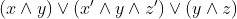=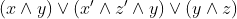[ Commutative property]

=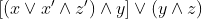=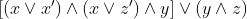=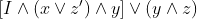[ I is the identity element]

=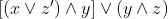=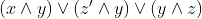=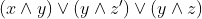[ Commutative property]

=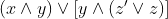=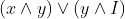=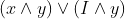=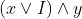=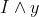=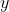#### Earn Coin

Coins can be redeemed for fabulous gifts.

Similar Homework Help Questions
• ### 7. (a) Find an example of a Boolean algebra with elements x, y, and z for which xty-x + z but yz. (b) Prove that in any Boolean algebra, if xy- z and+ yxz, then y -z 7. (a) Find an example of a...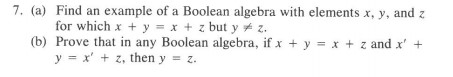7. (a) Find an example of a Boolean algebra with elements x, y, and z for which xty-x + z but yz. (b) Prove that in any Boolean algebra, if xy- z and+ yxz, then y -z 7. (a) Find an example of a Boolean algebra with elements x, y, and z for which xty-x + z but yz. (b) Prove that in any Boolean algebra, if xy- z and+ yxz, then y -z

• ### 8. (3 pts) Show the Boolean algebra steps to simplify the following: F = X'. Y'...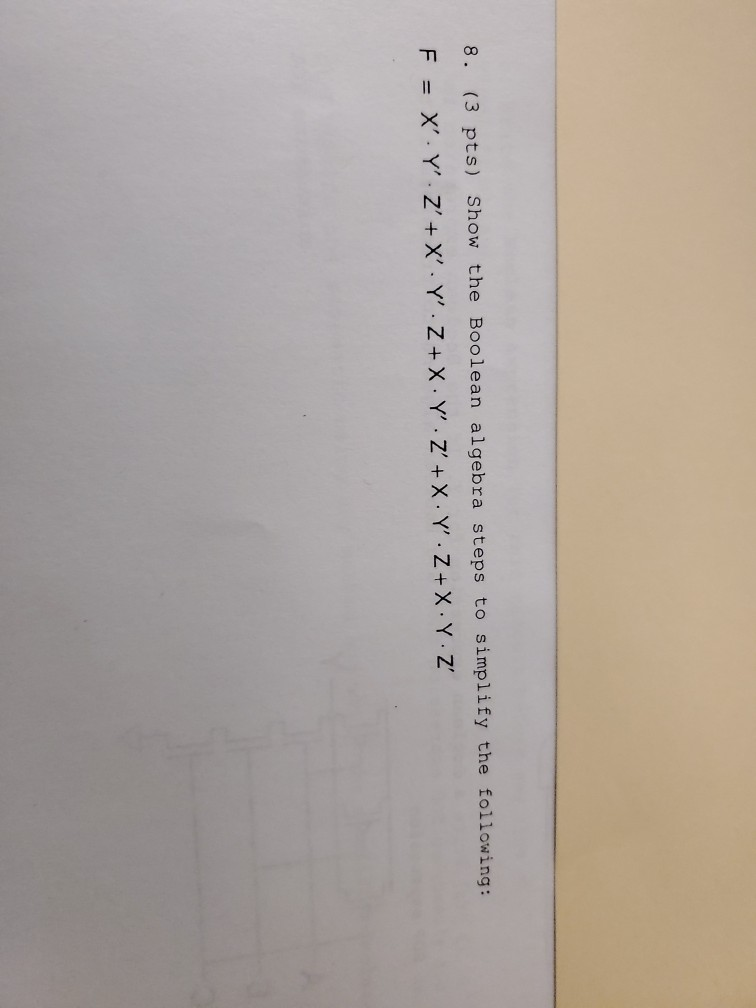8. (3 pts) Show the Boolean algebra steps to simplify the following: F = X'. Y' Z' + X Y '. Z+X Y' Z' + X Y Z+X Y Z

• ### Q2: 1. Proof this Boolean expression. Use Boolean Algebra (X+Y). (Z+W).(X'+Y+W) = Y.Z+X.W+Y.W 2. For this...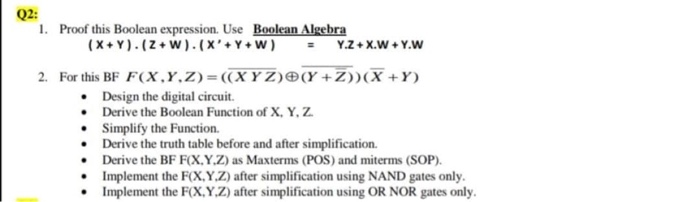Q2: 1. Proof this Boolean expression. Use Boolean Algebra (X+Y). (Z+W).(X'+Y+W) = Y.Z+X.W+Y.W 2. For this BF F(X,,Z)=((XYZ)(X +Z))(X+Y) • Design the digital circuit Derive the Boolean Function of X, Y, Z. Simplify the Function Derive the truth table before and after simplification. Derive the BF F(X,Y,Z) as Maxterms (POS) and miterms (SOP). Implement the F(X,Y,Z) after simplification using NAND gates only. Implement the F(X,Y,Z) after simplification using OR NOR gates only.

• ### Consider the Boolean function        F1 = X' · Z + X ' · Y · Z...

Consider the Boolean function        F1 = X' · Z + X ' · Y · Z + X · Y ' + X · Y' · Z (a) Implement F1, in the form as given, using 2-input ANDs, 2-input ORs and NOT gates. How many gates did you use? (b) Simplify F1 using Boolean algebra identities. Show all the steps & the identities used at each step. (c) Implement the simplified form of F1 using 2-input ANDs, 2-input ORs and...

• ###  Using properties of Boolean algebra, simplify the following Boolean expressions so they could be built...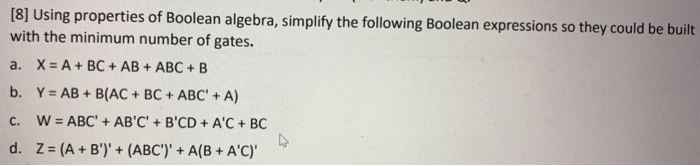Using properties of Boolean algebra, simplify the following Boolean expressions so they could be built with the minimum number of gates. a. X= A + BC + AB + ABC + B b. Y = AB + B(AC + BC + ABC' + A) C. W = ABC' + AB'C' + B'CD + A'C + BC d. Z = (A + B')' + (ABC')' +A(B + A'C)'

• ### Simplify the following functional expression using Boolean algebra and its identities. List the identity used at...

Simplify the following functional expression using Boolean algebra and its identities. List the identity used at each step. x(y+z)(x'+z')

• ### 1. Let B-(0, 1). Define x + y max(x, y) and x . y-min(x, y), and let the complement of x of be 1-x (ordinary subtraction). Show whether or not B forms a Boolean algebra under these operations. 2....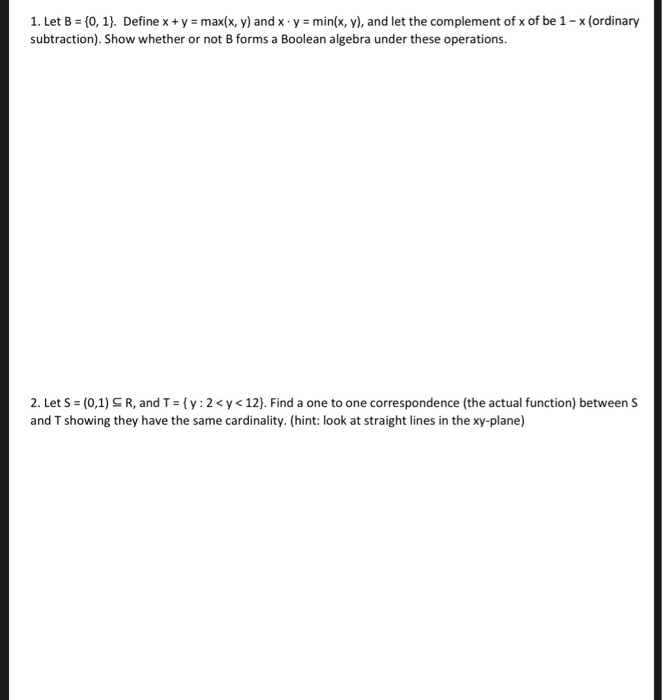1. Let B-(0, 1). Define x + y max(x, y) and x . y-min(x, y), and let the complement of x of be 1-x (ordinary subtraction). Show whether or not B forms a Boolean algebra under these operations. 2. Let S-(0,1 R, and T = { y : 2 < y < 12). Find a one to one correspondence (the actual function) between S and T showing they have the same cardinality. (hint: look at straight lines in the xy-plane)...

• ### simplify expression using theorems of boolean algebra Simplify expression using theorems of boolean algebra A middot...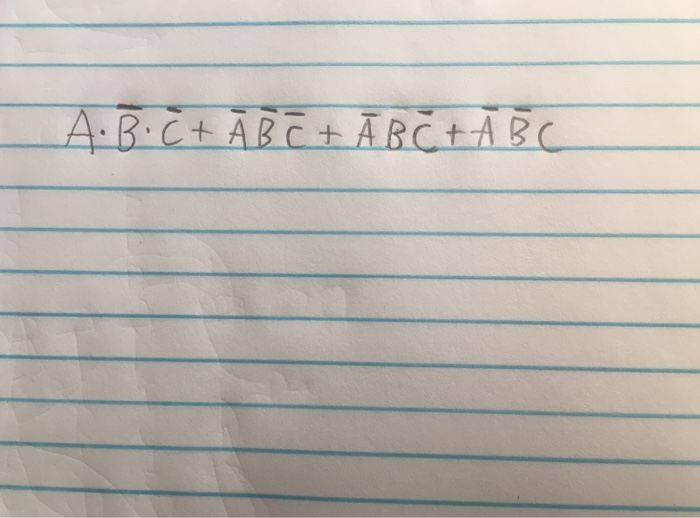simplify expression using theorems of boolean algebra Simplify expression using theorems of boolean algebra A middot B bar middot C bar + A bar B bar C bar + A bar BC bar + A bar B bar C

• ### Simplify the Boolean function F (x, y, z) lx +y) (x'+z) and implement with two-level NOR...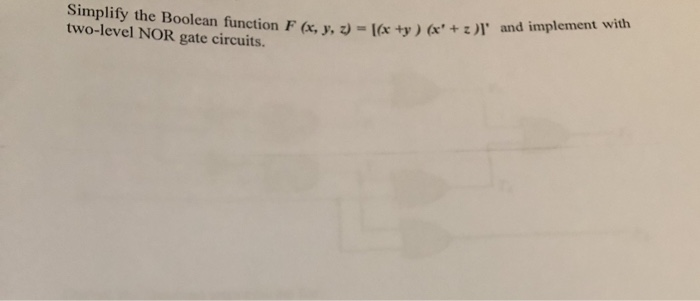Simplify the Boolean function F (x, y, z) lx +y) (x'+z) and implement with two-level NOR gate circuits.

• ### Use Boolean algebra to simplify. a = (NOT B) * (NOT C + NOT A) *...

Use Boolean algebra to simplify. a = (NOT B) * (NOT C + NOT A) * (NOT G + (NOT D + NOT A)) * (NOT H + ((NOT E + NOT A)*(NOT F + (NOT D + NOT A)))